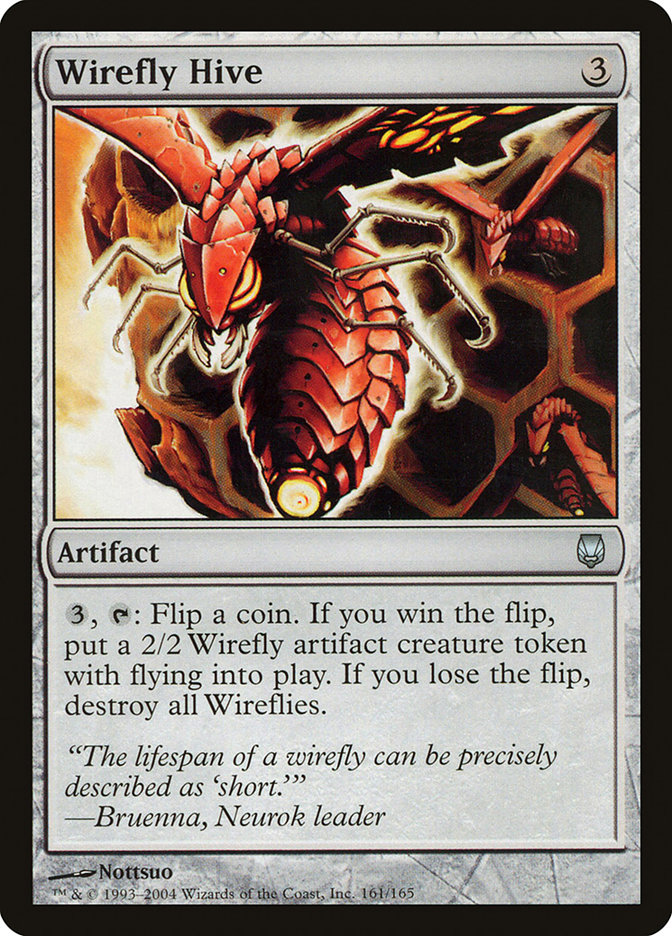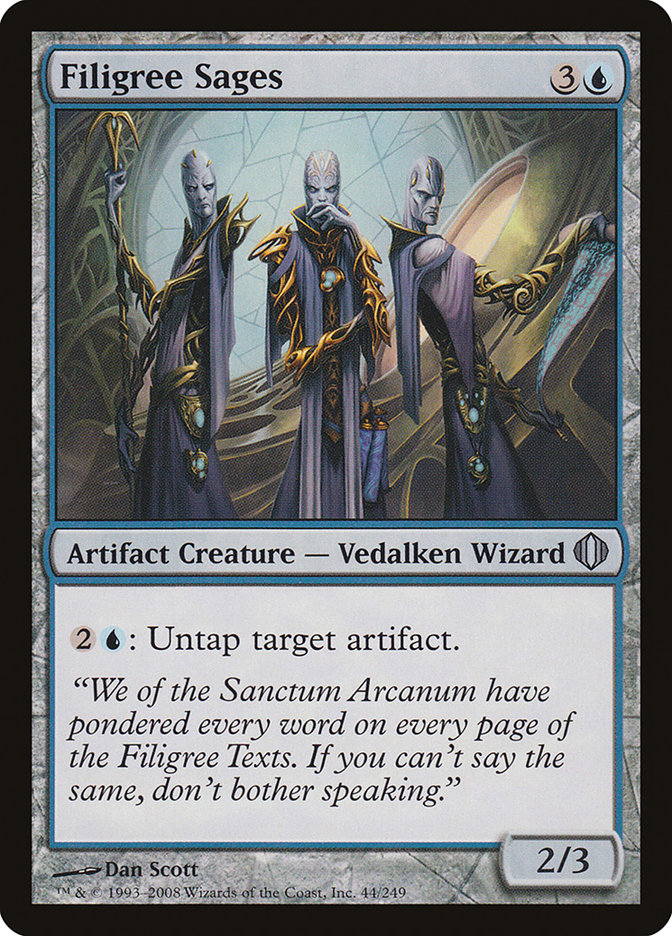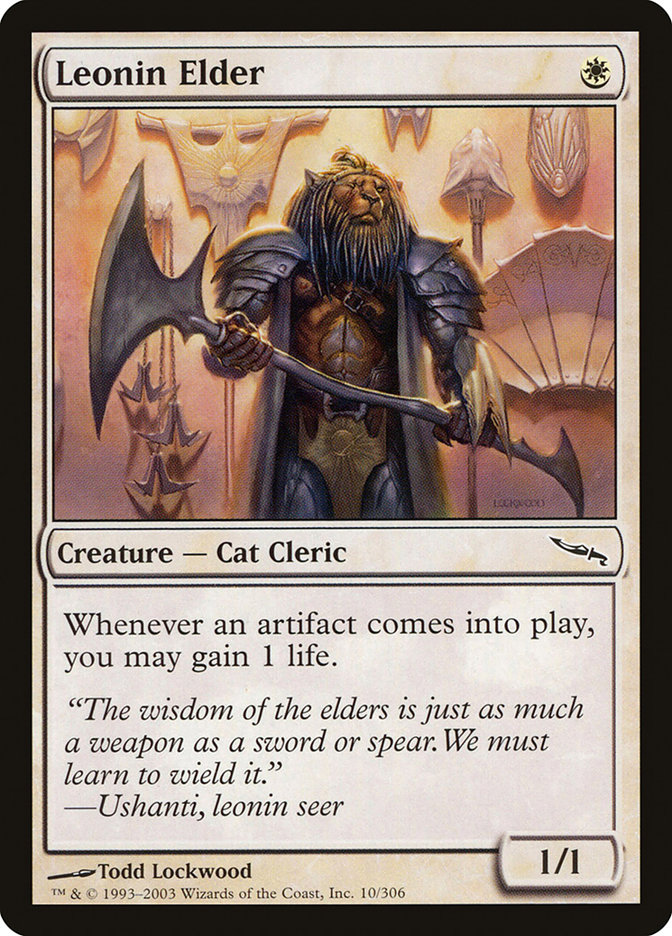# Wirefly Hive Problem

This puzzle comes from a video about Magic the Gathering that my brother sent me, which you can watch here.

The video is more about the specific rules of Magic, but this isn’t a Magic blog, so let’s get to the math as soon as possible.

I’ll try to summarize what’s going on here, but I don’t actually play Magic, so please forgive the inevitable inaccuracy here.

It’s your turn, and on your side of the board, you have some infinite source of mana, a Wirefly Hive, and Filigree Sages. Meanwhile, your opponent has a Leonin Elder.Basically, what this means is that, due to the effect of Filigree Sages, you can activate Wirefly Hive as many times as you want this turn. Each time you do so, you flip a coin.

• If it comes up heads, you get a Wirefly with 2 power (i.e attack strength), and your opponent gains 1 life from the effect of Leonin Elder.
• However, if it’s tails, all your Wireflies are destroyed. Plus, your opponent keeps their increased life!

This means that if you flip several heads in a row, you can gain a bunch of attack, potentially enough to kill your opponent. However, the more you flip tails, the harder your task becomes, as your opponent’s life steadily climbs higher.

The question is: if your opponent starts with $$L$$ life, what is the probability that you eventually amass enough Wireflies to win?

# Small $$L$$

Obviously if $$L = 0$$ you have already won, so let’s look at $$L = 1$$.

If we flip heads, then we get 1 Wirefly, and our opponent increases to 2 life. Because our Wirefly has attack 2, that’s enough for us to win.

But what if we flip tails?

All zero of our Wireflies get destroyed, and our opponent remains at 1 life, so this is actually a no-op. Nothing has happened, and we are free to keep flipping until we get a heads.

In other words, the probability of winning with $$L = 1$$ is 100%!

How about $$L = 2$$?

# Recurrence Relation

Just like before, we can keep flipping tails at the start, and nothing will happen. So eventually, we will flip a head, and get one Wirefly, bringing our opponent to 3 life. But now it gets harder.

• If we get another heads, we get a second Wirefly, and our opponent heals to 4 life. We can now attack with our two Wireflies for 4 damage and win.
• If we get tails, we lose our Wirefly, and have to start all over, but our opponent starts with 3 life this time.

If we let $$p(L)$$ be the probability of winning, when our opponent starts with $$L$$ life, then we have

$$p(2) = \frac{1}{2} + \frac{1}{2} p(3)$$

We can do something similar for higher $$L$$. If our opponent starts with $$L$$ life, then we flip the coin until we get our first head, giving our opponent $$L+1$$ life, and us one Wirefly. After that, if we get a streak of $$L$$ heads, our opponent will have $$2L$$ life, but we will have $$L$$ Wireflies total, and that is just enough to win. However, if our streak only lasts $$k < L$$ heads, we have to start over, with our opponent at $$L+k$$ life.

The probability of getting a streak of $$L$$ heads (remember, the first is “free”), is $$1/2^{L-1}$$. The probability of getting $$k$$ heads followed by a tail is $$1/2^{k-1+1}$$. Putting it all together, we get:

$$p(L) = \frac{1}{2^L} + \sum_{k = 1}^{L} \frac{1}{2^k} p(L+k)$$

Unlike normal recurrence relations, this grows towards higher values of $$L$$, and we have no base case to start with (try expanding this formula for $$L=1$$!).

Additionally, this relation is under-determined. For example, it is satisfied by $$p(L) = 1$$ always, which could be the answer, but as we discover below, it is not. So we’ve lost some information somewhere, but I don’t know what it is.

I was not able to get anywhere with this, but maybe there’s some fancy generating function tricks one can do.

# Random Walk

To make some progress, let’s transform the problem a bit.

If instead, we weaken our Wireflies to have only 1 attack, and change the effect on Leonin Elder to be “Whenever an artifact is destroyed, gain 1 life”, then we get the same puzzle, but it’s easier to reason about geometrically!

(If our opponent has $$L$$ life, we still have to flip $$L$$ heads in a row to get lethal damage. And when we break a streak of $$k$$ heads, we still have to start over with our opponent at $$L+k$$ life.)

Let’s draw a grid, where the x-axis is “opponent’s life”, and the y-axis is “number of Wireflies”. Each time we flip heads, we move up one step, and when we flip tails, we slide diagonally down to the x-axis. Our goal is to touch the diagonal $$x=y$$, at which point we have lethal damage.Now our problem takes the form of a random walk!

In order to figure out the probability of touching the diagonal, let’s figure out the probability of touching it at each point.

If our opponent starts with $$L$$ life, and we touch the diagonal at $$(N, N)$$, then we must have:

• Traversed some path from $$(L, 0)$$ to $$(N, 0)$$, without touching the diagonal.
• Then we flipped $$N$$ consecutive heads.

The probability of the latter is easy to compute; we’ve done it several times already: $$1/2^{N-1}$$. The former is trickier.

Any particular path from $$(L, 0)$$ to $$(N, 0)$$ comes from a specific sequence of heads streaks, punctuated by arbitrary runs of tails. For example, the sequence shown in the diagram above has streaks of $$(1, 2, 1)$$, and could have been produced by:

• HTHHTHT
• HTTTTHHTTTTTHTTTTTT
• TTTTTHTHHTTHT
• and so on

What’s the probability of getting this particular sequence?

• We have to get a streak of one head: $$1/2$$
• Then a streak of two heads: $$1/4$$
• And lastly, a streak of one head again: $$1/2$$

In total, that’s $$1/16$$.

In general, the probability of getting a streak pattern of $$(k_1, k_2, \ldots, k_i)$$ is $$1/2^{k_1} \cdot 1/2^{k_2} \cdots 1/2^{k_i}$$, or in other words, $$1/2^{k_1 + k_2 + \cdots + k_i}$$. And since we know we need $$N - L$$ heads to get from $$(L, 0)$$ to $$(N, 0)$$, that means each path has the exact same probability: $$1/2^{N - L}$$.

The only thing we’re missing is the number of paths from $$(L, 0)$$ to $$(N, 0)$$. If we call that quantity $$a_{L, N}$$, then the probability that we win is:

$$p(L) = \sum_{N = L}^\infty p(\textrm{walked to (N, 0)}) \cdot \frac{1}{2^{N-1}} = \sum_{N = L}^\infty \frac{a_{L, N}}{2^{N - L}} \cdot \frac{1}{2^{N-1}}$$

The only thing left to do is find the values of $$a_{L, N}$$.

# Counting Paths

Counting these paths turns out to be quite an ordeal, and I never ended up finding a closed form for them. Still though, there’s a clean recurrence relation that one can use to compute these things, so let’s dive in.

If we’re looking for a path from $$(L, 0)$$ to $$(N, 0)$$, our last step has to be a diagonal. There’s only a few possible places we can slide down the diagonal and hit $$(N, 0)$$.Specifically, we can be on any point $$(N - i, N + i)$$, as long as $$N - i < N + i$$, otherwise we’d be on (or past) the diagonal, and this would not be a valid path.

The only way to get from $$(L, 0)$$ to $$(N - i, N + i)$$ is to make it to $$(N - i, 0)$$, and then go straight up. So each path to $$(N - i, 0)$$ gives us exactly one path to $$(N, 0)$$, meaning the number of paths is just:

$$a_{L, N} = \sum_{i} a_{L, N - i}$$

where the sum is taken over all $$i$$ such that $$N - i < N + i$$. Also, we can skip all $$i$$ such that $$N - i < L$$, because there are no paths going backwards.

For a specific example, consider $$a_{2, 6}$$.

We can slide down to $$(6, 0)$$ from $$(5, 1)$$ or $$(4, 2)$$, but not $$(3, 3)$$. So we have that $$a_{2, 6} = a_{2, 5} + a_{2, 4}$$. The possible paths are shown below, albeit, kind of cluttered:We see that there are 3 possible paths, two of which come from $$a_{2, 5}$$ (blue and purple), and one of which comes from $$a_{2, 4}$$ (cyan).

# Simpler Recurrence

This is a pretty simple recurrence relation, but we can actually do better!

Consider $$a_{2, 7}$$. From our existing knowledge, we have that $$a_{2, 7} = a_{2, 6} + a_{2, 5} + a_{2, 4}$$. But if we replace $$a_{2, 5} + a_{2, 4}$$, then we get that $$a_{2, 7} = 2 a_{2, 6}$$!

Similarly, $$a_{2, 8} = a_{2, 7} + a_{2, 6} + a_{2, 5}$$, but here we are not quite so lucky – we do not have all the terms we need. The best we can do is $$a_{2, 8} = 2 a_{2, 7} - a_{2, 4}$$.

And in general, for all $$n$$ and $$k > n$$, we have:

$$a_{n, 2k+1} = 2 a_{n, 2k}$$
$$a_{n, 2k} = 2 a_{n, 2k-1} - a_{n, k}$$

# Conclusion?

Unfortunately, I was not able to find any closed-form solution here.

I threw the recurrence relation into Python, and got the following results:

$$L$$ $$p(L)$$
0 N/A
1 1
2 0.68332
3 0.36663
4 0.18645
5 0.093619
6 0.046859
7 0.023435
8 0.011718
9 0.0058593
10 0.0029297

Additionally, I found an OEIS sequence that counts $$a_{2, n}$$, and I was able to find other references to this sequence, but not the specific sum we’re looking for. Wolfram Alpha doesn’t produce any reasonable guesses for a closed form either. Can’t win them all, I guess.